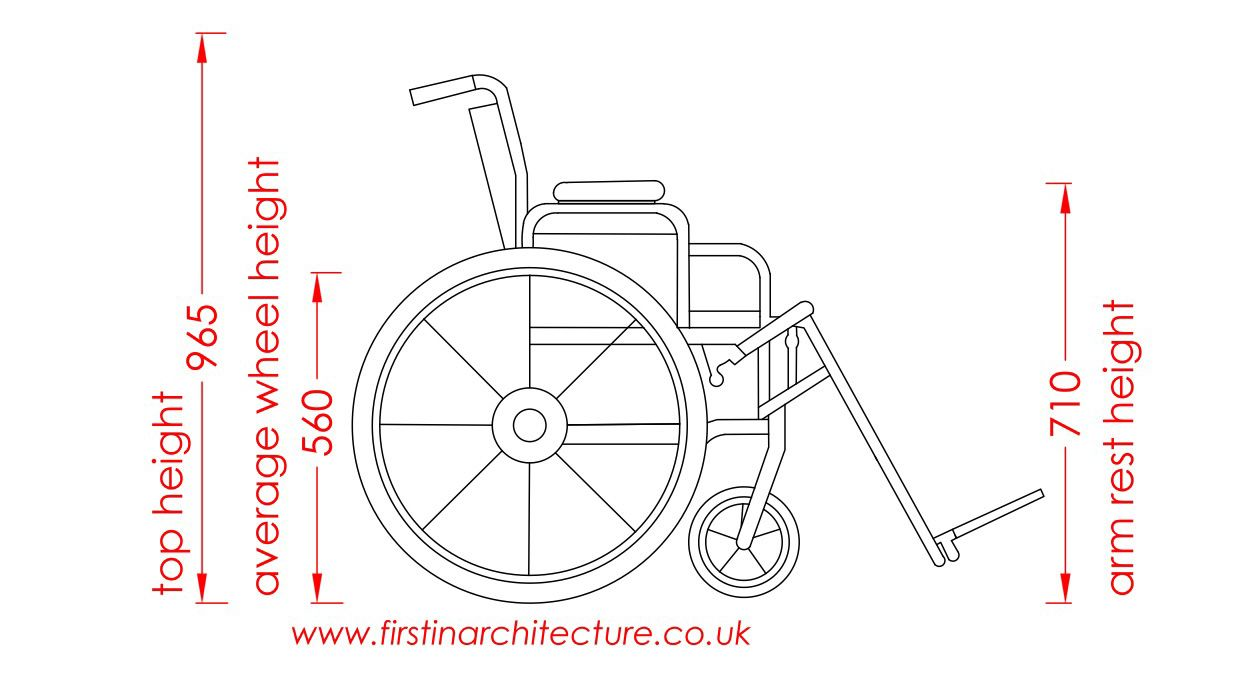Autonomous Wheelchair

# Wheelchair Calculations §

Top Motor candidates:

Power?

• ?

So concluding that we need about 300W. If it tries to go up the ramp, it is going to start overhead.

300W = V * A

The voltage is fixed, that determines the RPM. Actually, we need to know the power needed to move the body.

When it comes to designing the gearbox at the end, this is going to be very important: what sorts of gear reduction are we looking at?

Assumptions

VariableValueNotes
Gross Weight 116kg100kg + 16kg = 116kg
Rolling Resistance coefficient 0.002Bicycle tire on concrete
Time to accelerate 1s
Maximum Speed ~3m/ssource , 12km/h demonstration at 4:48
Maximum slope angle 30degthe maximum angle at which the wheelchair can move out
VariableValueNotes
Wheel radius 28cmThis is an estimateFirst, let’s see how much force we need to move this wheelchair without acceleration, which is simply given by the following equation.